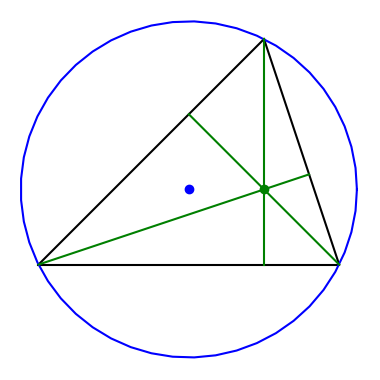# Relating circumcenter and orthocenterThe previous post mentioned that the law of sines gives you the diameter of a circle through the vertices of a triangle. How would you find the center of this circle, the blue dot in the image above?

If the angles of the triangle are α. β, and γ, then the trilinear coordinates of the circumcenter, the center of the circumscribed circle, are

cos α : cos β : cos γ.

Trilinear coordinates are written with colons because the are proportions; any multiple of the same three numbers corresponds to the same point.

My earlier post on the nine-point circle mentioned the orthocenter of a triangle, the point where the three altitudes of the triangle intersect. This is the green dot in the image above. The orthocenter has trilinear coordinates

sec α : sec β : sec γ.

That is, the trilinear coordinates of the orthocenter are the reciprocals of the trilinear coordinates of the circumcenter.

Another way to state this is to say that the orthocenter and circumcenter are isogonal conjugates. Isogonal conjugation can be defined in terms of reflections, but the simplest description is in terms of trilinear coordinates.